### Home > CALC > Chapter 2 > Lesson 2.3.1 > Problem2-110

2-110.
1. Lena loves limits! She wants to find a shortcut for limits so she can predict a function's value without graphing. Use the following limits to look for a shortcut and explain to Lena how she can find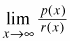without graphing. (Be careful! The expressions below are all slightly different.) Homework Help ✎

1.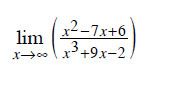2.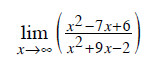3.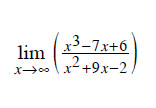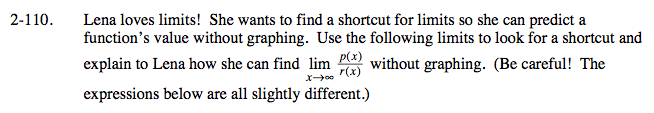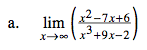Compare the highest power in the numerator and the denominator small exponent (x²) on top large exponent (x³) on the bottom.

$\lim_{x\rightarrow \infty }\frac{x^{2}}{x^{3}}=\lim_{x\rightarrow \infty }\frac{1}{x}$

0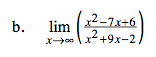As x→∞, only the highest power of the numerator and denominator is powerful enough to affect the end behavior of a function.

$\lim_{x\rightarrow \infty }\frac{x^{2}}{x^{2}}=$

1$\lim_{x\rightarrow \infty }\frac{x^{3}}{x^{2}}=\lim_{x\rightarrow \infty }x=$

Limit Does Not Exist but y → ∞